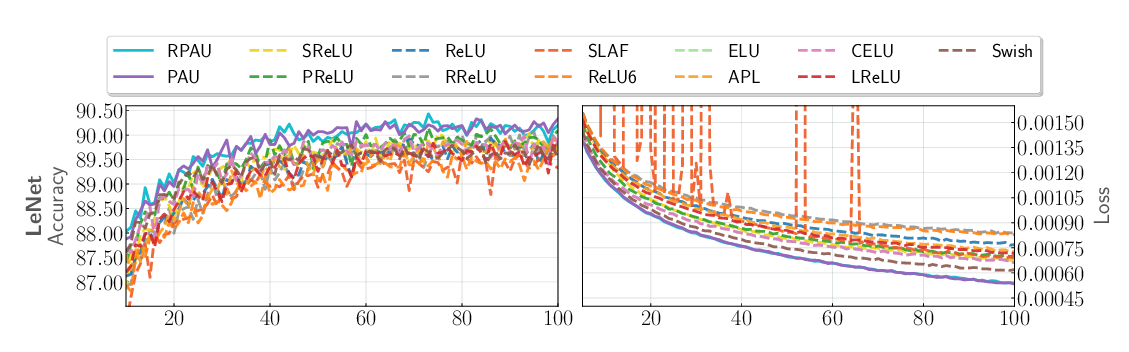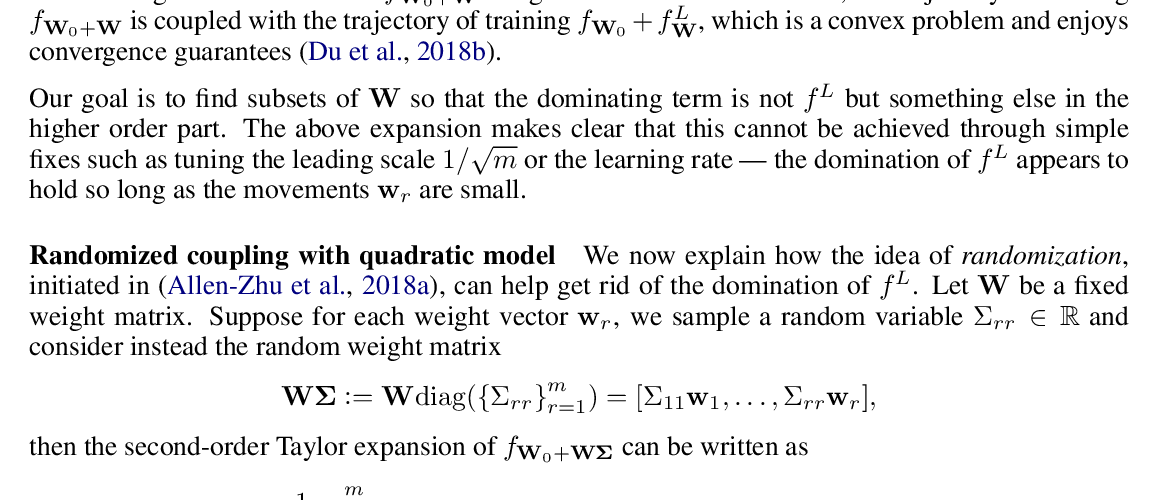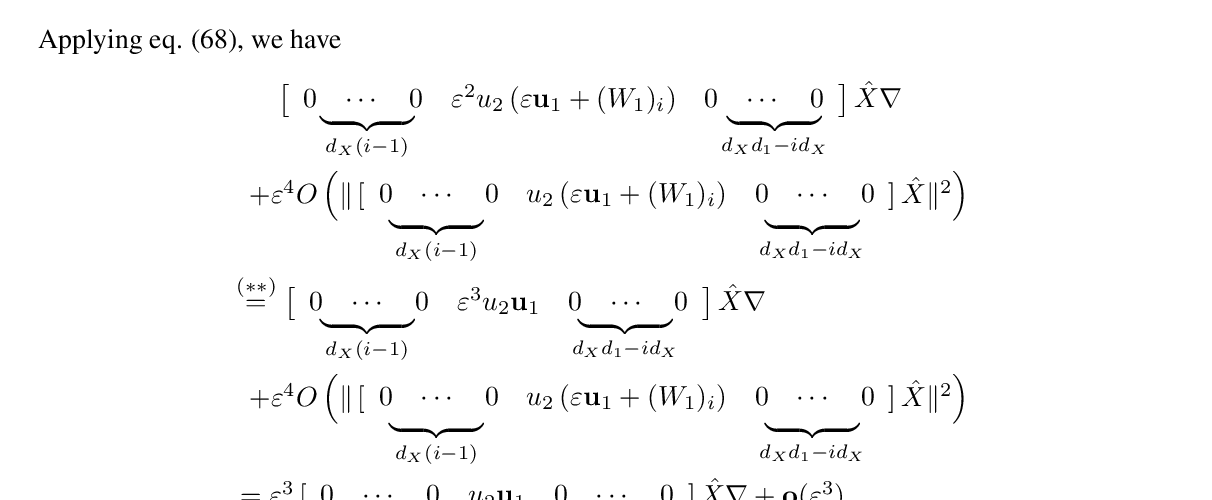## Effect of Activation Functions on the Training of Overparametrized Neural Nets

### Abhishek Panigrahi, Abhishek Shetty, Navin Goyal

Keywords: deep learning theory

Abstract: It is well-known that overparametrized neural networks trained using gradient based methods quickly achieve small training error with appropriate hyperparameter settings. Recent papers have proved this statement theoretically for highly overparametrized networks under reasonable assumptions. These results either assume that the activation function is ReLU or they depend on the minimum eigenvalue of a certain Gram matrix. In the latter case, existing works only prove that this minimum eigenvalue is non-zero and do not provide quantitative bounds which require that this eigenvalue be large. Empirically, a number of alternative activation functions have been proposed which tend to perform better than ReLU at least in some settings but no clear understanding has emerged. This state of affairs underscores the importance of theoretically understanding the impact of activation functions on training. In the present paper, we provide theoretical results about the effect of activation function on the training of highly overparametrized 2-layer neural networks. A crucial property that governs the performance of an activation is whether or not it is smooth: • For non-smooth activations such as ReLU, SELU, ELU, which are not smooth because there is a point where either the ﬁrst order or second order derivative is discontinuous, all eigenvalues of the associated Gram matrix are large under minimal assumptions on the data. • For smooth activations such as tanh, swish, polynomial, which have derivatives of all orders at all points, the situation is more complex: if the subspace spanned by the data has small dimension then the minimum eigenvalue of the Gram matrix can be small leading to slow training. But if the dimension is large and the data satisﬁes another mild condition, then the eigenvalues are large. If we allow deep networks, then the small data dimension is not a limitation provided that the depth is sufﬁcient. We discuss a number of extensions and applications of these results.

## Similar Papers

##### Padé Activation Units: End-to-end Learning of Flexible Activation Functions in Deep Networks
###### Alejandro Molina, Patrick Schramowski, Kristian Kersting,##### Beyond Linearization: On Quadratic and Higher-Order Approximation of Wide Neural Networks
###### Yu Bai, Jason D. Lee,##### Piecewise linear activations substantially shape the loss surfaces of neural networks
###### Fengxiang He, Bohan Wang, Dacheng Tao,